7.1 Transition Rates according to the NMP Theory

The transition rates of charge transfer reactions must be discussed on the basis of configuration coordinate diagrams. One such a diagram is depicted in Fig. 7.1 for the case of hole trapping. The adiabatic potentials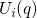and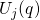in the configuration coordinate diagrams are approximated by the parabolas around their respective minima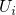and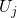assuming the harmonic approximation: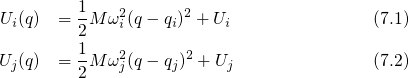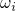and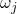denote the vibrational frequency of the oscillator potential when the defect is in the charge stateand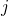, respectively. It is stressed that these oscillator potentials of both charge states are assumed to have different curvatures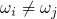in this derivation. The transition barriers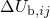and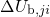differ by the energy, which can be expressed asusing the relations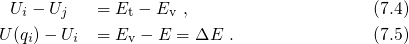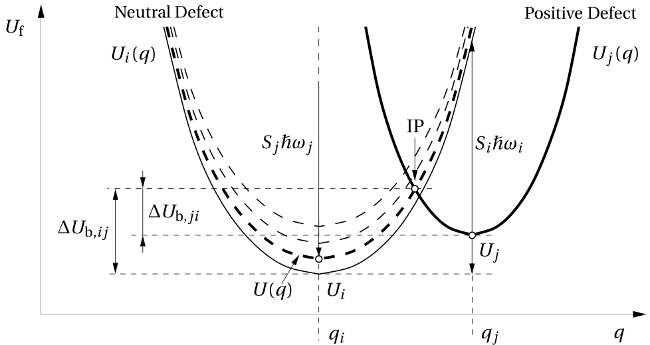Figure 7.1: The configuration coordinate diagram for a hole trapping process. The left parabola corresponds to the case when the defect is neutral and the holes reside in the substrate valence band. Then the hole can be thermally excited by an energy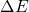, accompanied by an upwards shift of the left parabola from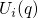(solid) to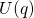(dashed). By contrast, when the defect is positively charged (right parabola), the whole system including the defect and the substrate is represented by the parabola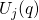(solid). In general, the curvature ofanddo not need to be equal. As a consequence, both adiabatic potentials are characterized by their own oscillator frequency (,) and in further consequence their own Huang Rhys factor (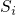,).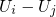corresponds to the separation of the trap level from the valence band edge and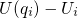gives the kinetic energy of the substrate hole. Making use of expression (7.3), the difference between the transition barriers can be expressed as: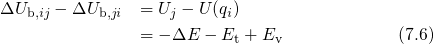Using the identity (2.62) and equation (7.6), one obtains the following relation: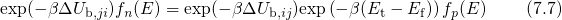Analogously to the equation (2.59), the trapping dynamics are governed by the rate equation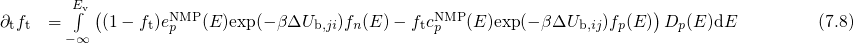with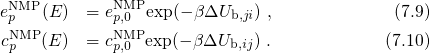Making use of (7.6), the above rate equation can be simplified to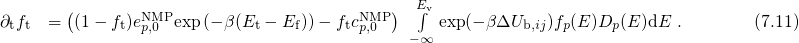In order to evaluate the integral in the above equation, analytic expressions for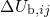and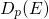are required. The former is defined as the energy difference betweenand the intersection point IP in the configuration coordinate diagram. The position of of this point can be derived from the condition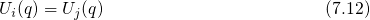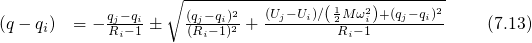with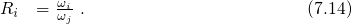Inserting the expression (7.13) into equation (7.1), one obtains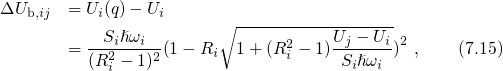where the Huang Rhys factoris defined by the equation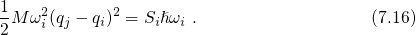A second order expansion of equation (7.15) delivers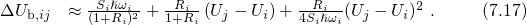According to equation (7.14), the oscillator frequenciesanddiffer and thus the quantity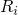deviates from unity. Sinceenters the above expression for the barrier height, the oscillator frequencies have a strong impact on the transition rates. When the kinetic energy of the substrate hole is taken into account,must be replaced by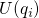and equation (7.17) can be rewritten as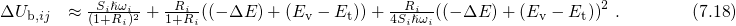In the case of strong electron-phonon coupling,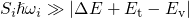holds and the third term can be neglected. Assuming parabolic bands (see Appendix A.4), the valence band density of states can be expressed as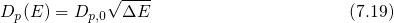with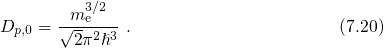Using (7.19), the integral in equation (7.11) can be evaluated as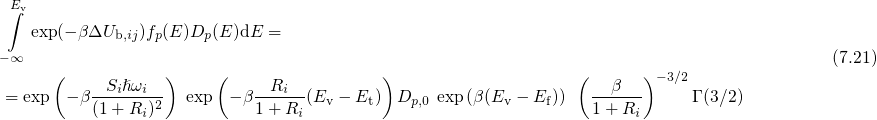and simplifies to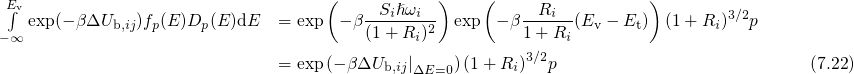with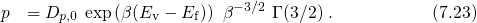Here,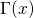denotes the Gamma function, which is defined by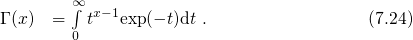With (7.22), the compact form of the rate equation (7.11) can be rewritten as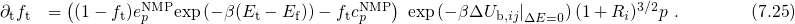From this, it follows that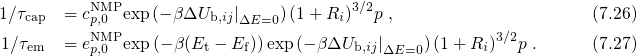Just as in the standard SRH theory (see Section 2.5), the right-hand side of equation (7.26) can be simplified using the definition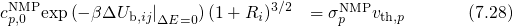with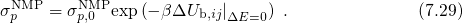Analogously to Section 2.5, thermal equilibrium can be assumed so that the trap occupation follows Fermi-Dirac statistics and detailed balance applies for the rate equation (7.25). Then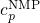equals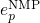and the hole capture and emission time constant reads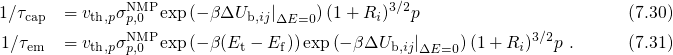It is emphasized that the barrier heights are correctly calculated by determining the crossing point of two parabolas. Thereby, one avoids the artificial differentiation, whetheris located above or below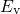, as it has been the case in equation (6.10) of the TSM. Additionally, the NMP barriers have not been assumed to be independent of the energy of the hole in contrast to the Kirton model and the TSM.